# Intrinsic Semiconductor

Semiconductor is a material whose conductivity lies in-between that of the conductors and the insulators. Semiconductors which are chemically pure, meaning free of impurities, are called Intrinsic Semiconductors or Undoped Semiconductor or i-type Semiconductor. The most common intrinsic semiconductors are Silicon (Si) and Germanium (Ge), which belong to Group IV of the periodic table. The atomic numbers of Si and Ge are 14 and 32, which yields their electronic configuration as 1s2 2s2 2p6 3s2 3p2 and 1s2 2s2 2p6 3s2 3p6 4s2 3d10 4p2, respectively.This indicates that both Si and Ge have four electrons each in their outer-most i.e. valence shell (indicated by red colour). These electrons are called valence electrons and are responsible for the conduction-properties of the semiconductors.

The crystal lattice of Silicon (it is the same even for Germanium) in two-dimension is as shown in Figure 1. Here it is seen that each valence electron of a Si atom pair with the valence electron of the adjacent Si atom to form a covalent bond.

After pairing, the intrinsic semiconductor becomes deprived of free charge carriers which are nothing but the valence electrons. Hence, at 0K the valence band will be full of electrons while the conduction band will be empty (Figure 2a). At this stage, no electron in the valence band would gain enough energy to cross the forbidden energy gap of the semiconductor material. Thus the intrinsic semiconductors act as insulators at 0K.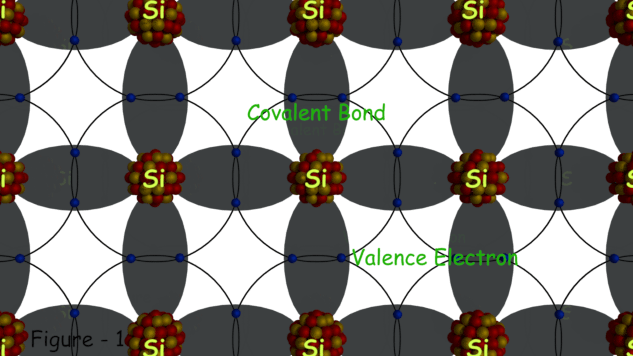However, at room temperature, the thermal energy may cause a few of the covalent bonds to break, thus generating the free electrons as shown in Figure 3a. The electrons thus generated get excited and move into the conduction band from the valence band, overcoming the energy barrier (Figure 2b). During this process, each electron leaves behind a hole in the valence band. The electrons and holes created in this way are called intrinsic charge carriers and are responsible for the conductive properties exhibited by the intrinsic semiconductor material.

Although the intrinsic semiconductors are capable of conducting at room temperature, here we can note that the conductivity so exhibited is low as there are only a few charge carriers. But as the temperature increases, more and more covalent bonds break which results in more and more number of free electrons. The number of free electrons, in turn, results in the movement of a greater number of electrons into the conduction band from the valence band. As the population of the electrons in the conduction band increases, the conductivity of the intrinsic semiconductor also increases. However, the number of electrons (ni) in the intrinsic semiconductor remains always equal to the number of holes in it (pi).

On applying an electric field to such an intrinsic semiconductor, the electron-hole pairs can be made to drift under its influence. In this case, the electrons move in the direction opposite to that of the applied field while the holes move in the direction of the electric field as shown in Figure 3b. This means that the direction along which the electrons and the holes move are mutually opposite. This is because, as an electron of a particular atom moves towards say, left, by leaving a hole in its place, the electron from the neighboring atom occupies its place by recombining with that hole. However while doing so, it would have left one more hole in its place. This can be viewed as the movement of the holes (towards right side in this case) in the semiconductor material. These two movements, although opposite in direction, result in the total flow of current through the semiconductor.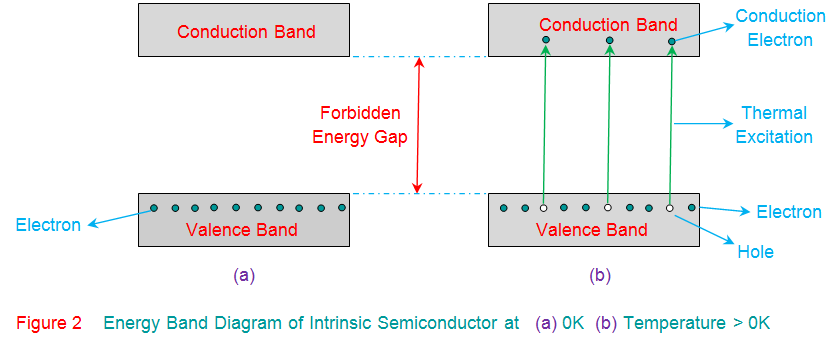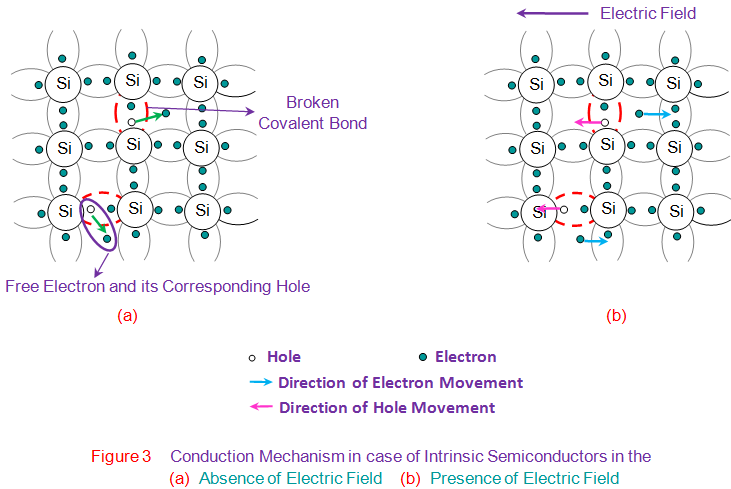Mathematically the charge carrier densities in intrinsic semiconductors are given by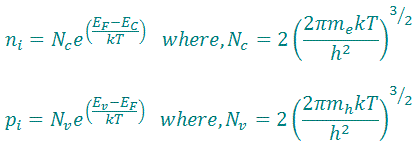Here,
Nc is the effective densities of states in the conduction band.
Nv is the effective densities of states in the valence band.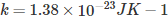is the Boltzmann constant.
T is the temperature.
EF is the Fermi energy.
Ev indicates the level of valence band.
Ec indicates the level of conduction band.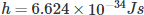is the Planck constant.
mh is the effective mass of a hole.
me is the effective mass of an electron.

Want To Learn Faster? 🎓
Get electrical articles delivered to your inbox every week.
No credit card required—it’s 100% free.